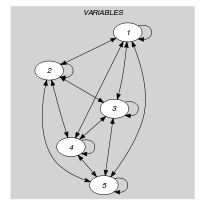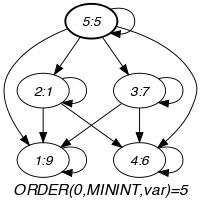## 5.250. maximum_modulo

Origin
Constraint

$\mathrm{𝚖𝚊𝚡𝚒𝚖𝚞𝚖}_\mathrm{𝚖𝚘𝚍𝚞𝚕𝚘}\left(\mathrm{𝙼𝙰𝚇},\mathrm{𝚅𝙰𝚁𝙸𝙰𝙱𝙻𝙴𝚂},𝙼\right)$

Arguments
 $\mathrm{𝙼𝙰𝚇}$ $\mathrm{𝚍𝚟𝚊𝚛}$ $\mathrm{𝚅𝙰𝚁𝙸𝙰𝙱𝙻𝙴𝚂}$ $\mathrm{𝚌𝚘𝚕𝚕𝚎𝚌𝚝𝚒𝚘𝚗}\left(\mathrm{𝚟𝚊𝚛}-\mathrm{𝚍𝚟𝚊𝚛}\right)$ $𝙼$ $\mathrm{𝚒𝚗𝚝}$
Restrictions
 $|\mathrm{𝚅𝙰𝚁𝙸𝙰𝙱𝙻𝙴𝚂}|>0$ $𝙼>0$ $\mathrm{𝚛𝚎𝚚𝚞𝚒𝚛𝚎𝚍}$$\left(\mathrm{𝚅𝙰𝚁𝙸𝙰𝙱𝙻𝙴𝚂},\mathrm{𝚟𝚊𝚛}\right)$
Purpose

$\mathrm{𝙼𝙰𝚇}$ is a maximum value of the collection of domain variables $\mathrm{𝚅𝙰𝚁𝙸𝙰𝙱𝙻𝙴𝚂}$ according to the following partial ordering: $\left(X\mathrm{mod}𝙼\right)<\left(Y\mathrm{mod}𝙼\right)$.

Example
$\left(5,〈9,1,7,6,5〉,3\right)$

The $\mathrm{𝚖𝚊𝚡𝚒𝚖𝚞𝚖}_\mathrm{𝚖𝚘𝚍𝚞𝚕𝚘}$ constraint holds since its first argument $\mathrm{𝙼𝙰𝚇}$ is set to value 5, where $5\mathrm{mod}3=2$ is greater than or equal to all the expressions $9\mathrm{mod}3=0$, $1\mathrm{mod}3=1$, $7\mathrm{mod}3=1$ and $6\mathrm{mod}3=0$.

Typical
 $𝙼>1$ $𝙼<$$\mathrm{𝚖𝚊𝚡𝚟𝚊𝚕}$$\left(\mathrm{𝚅𝙰𝚁𝙸𝙰𝙱𝙻𝙴𝚂}.\mathrm{𝚟𝚊𝚛}\right)$ $|\mathrm{𝚅𝙰𝚁𝙸𝙰𝙱𝙻𝙴𝚂}|>1$ $\mathrm{𝚛𝚊𝚗𝚐𝚎}$$\left(\mathrm{𝚅𝙰𝚁𝙸𝙰𝙱𝙻𝙴𝚂}.\mathrm{𝚟𝚊𝚛}\right)>1$
Symmetry

Items of $\mathrm{𝚅𝙰𝚁𝙸𝙰𝙱𝙻𝙴𝚂}$ are permutable.

Arg. properties

Functional dependency: $\mathrm{𝙼𝙰𝚇}$ determined by $\mathrm{𝚅𝙰𝚁𝙸𝙰𝙱𝙻𝙴𝚂}$ and $𝙼$.

specialisation: $\mathrm{𝚖𝚊𝚡𝚒𝚖𝚞𝚖}$ ($\mathrm{𝚟𝚊𝚛𝚒𝚊𝚋𝚕𝚎}\mathrm{mod}\mathrm{𝚌𝚘𝚗𝚜𝚝𝚊𝚗𝚝}$ replaced by $\mathrm{𝚟𝚊𝚛𝚒𝚊𝚋𝚕𝚎}$).

Keywords
Arc input(s)

$\mathrm{𝚅𝙰𝚁𝙸𝙰𝙱𝙻𝙴𝚂}$

Arc generator
$\mathrm{𝐶𝐿𝐼𝑄𝑈𝐸}$$↦\mathrm{𝚌𝚘𝚕𝚕𝚎𝚌𝚝𝚒𝚘𝚗}\left(\mathrm{𝚟𝚊𝚛𝚒𝚊𝚋𝚕𝚎𝚜}\mathtt{1},\mathrm{𝚟𝚊𝚛𝚒𝚊𝚋𝚕𝚎𝚜}\mathtt{2}\right)$

Arc arity
Arc constraint(s)
$\bigvee \left(\begin{array}{c}\mathrm{𝚟𝚊𝚛𝚒𝚊𝚋𝚕𝚎𝚜}\mathtt{1}.\mathrm{𝚔𝚎𝚢}=\mathrm{𝚟𝚊𝚛𝚒𝚊𝚋𝚕𝚎𝚜}\mathtt{2}.\mathrm{𝚔𝚎𝚢},\hfill \\ \mathrm{𝚟𝚊𝚛𝚒𝚊𝚋𝚕𝚎𝚜}\mathtt{1}.\mathrm{𝚟𝚊𝚛}\mathrm{mod}𝙼>\mathrm{𝚟𝚊𝚛𝚒𝚊𝚋𝚕𝚎𝚜}\mathtt{2}.\mathrm{𝚟𝚊𝚛}\mathrm{mod}𝙼\hfill \end{array}\right)$
Graph property(ies)
$\mathrm{𝐎𝐑𝐃𝐄𝐑}$$\left(0,\mathrm{𝙼𝙸𝙽𝙸𝙽𝚃},\mathrm{𝚟𝚊𝚛}\right)=\mathrm{𝙼𝙰𝚇}$

Graph model

Parts (A) and (B) of Figure 5.250.1 respectively show the initial and final graph associated with the Example slot. Since we use the $\mathrm{𝐎𝐑𝐃𝐄𝐑}$ graph property, the vertex of rank 0 (without considering the loops) of the final graph is outlined with a thick circle.

##### Figure 5.250.1. Initial and final graph of the $\mathrm{𝚖𝚊𝚡𝚒𝚖𝚞𝚖}_\mathrm{𝚖𝚘𝚍𝚞𝚕𝚘}$ constraint(a) (b)